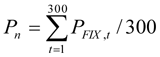# Rules

The Exchange computes Fixings based on the orders (20 best bids and 20 best asks) directed to all trading participants (indirect orders) and trades executed based on the indirect orders. The Fixing values are determined as per the following algorithm:

ORDERS

The second by second sections quotes are used to determine the value of the Fixing. The average price of indirect bids and asks that were entered by the trading members as at the moment n at which the Fixing is calculated.

Weighting coefficient decreases exponentially with increasing distance from the best quotes values. If no bids and/or asks are available at the time n at which the Exchange rate is calculated, the Pmid shall be deemed equal to the Pmid value at the moment n-1.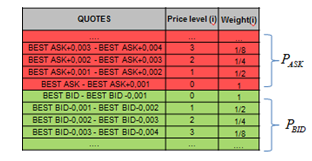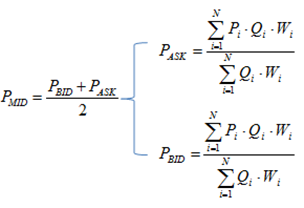DEALS

Every second the weighted by volume average price of transactions Pdeal is calculated. If no trades were executed over the period t shall not be calculated and Pfix shall be deemed equal to Pmid.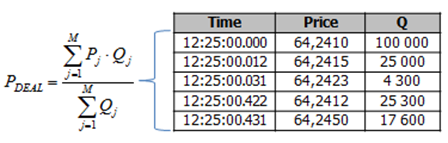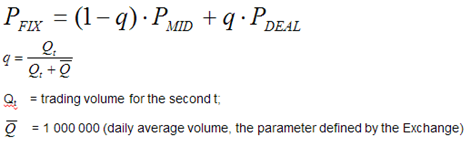FIXING

The Fixing is determined basing on the average values of trades and orders prices (Pfix) calculated every second from 12:25:01 pm through 12:30:00 pm MSK.